## DSP tutorial: RTTY decoder using IIR filters

This example uses IIR filters instead of FFTing chunks, it works well even on a noisy channel.

It uses the signal flow discussed in this paper: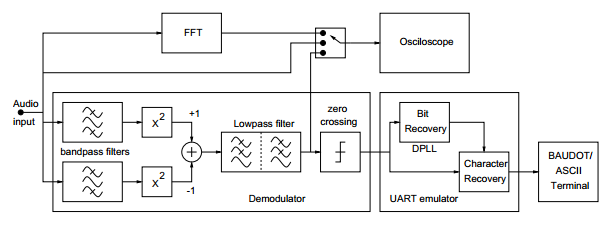Note that this example only implements the demodulator, UART emulator and Baudot terminal blocks from the diagram.

After the first bandpass filter you get the following signal on a clean channel: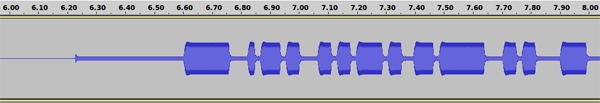On a noisy channel it looks like this: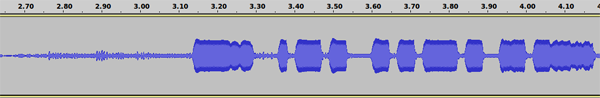After calculating the RMS (squaring the signal) on the clean channel: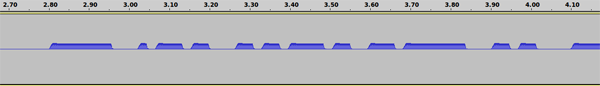On a noisy channel: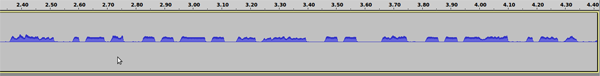After adding the two signal lines and lowpass filtering them on a clean channel: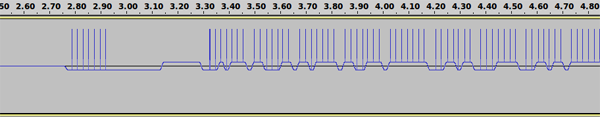On a noisy channel: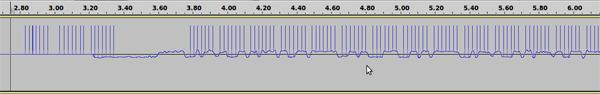The ticks mark the points where the DPLL algorithm returned (so that’s the point where the bit decision was made).

#### The DPLL algorithm

The DPLL algorithm is used for bit syncing, when it’s used, the bit decision can be made in the middle of a bit. The algorithm uses a counter called DPLLBitPhase. If it’s more than a bit’s length in samples, the function returns. The counter gets increased by every sample the DPLL reads from the demodulator input.

The sync correction works like this: if a bit change is detected, it checks if it happened at the half of one bit time. If the change was before the half, the DPLLBitPhase counter gets increased because it’s late, so the function will return earlier. If the change was after the half, the counter gets decreased, so the function will return later (reads more from the demodulator input before returning). The correction is only made once per DPLL function call.

#### Source code

 123456789101112131415161718192021222324252627282930313233343536373839404142434445464748495051525354555657585960616263646566676869707172737475767778798081828384858687888990919293949596979899100101102103104105106107108109110111112113114115116117118119120121122123124125126127128129130131132133134135136137138139140141142143144145146147148149150151152153154155156157158159160161162163164165166167 // for filter designing, see http://www-users.cs.york.ac.uk/~fisher/mkfilter/     // order 2 Butterworth, freqs: 865-965 Hz     public double bandPassFreq0(double sampleIn) {         xvBP0 = xvBP0; xvBP0 = xvBP0; xvBP0 = xvBP0; xvBP0 = xvBP0;         xvBP0 = sampleIn / 2.356080041e+04;         yvBP0 = yvBP0; yvBP0 = yvBP0; yvBP0 = yvBP0; yvBP0 = yvBP0;         yvBP0 = (xvBP0 + xvBP0) - 2 * xvBP0                     + (-0.9816582826 * yvBP0) + (3.9166274264 * yvBP0)                     + (-5.8882201843 * yvBP0) + (3.9530488323 * yvBP0);         return yvBP0;     }     // order 2 Butterworth, freqs: 1035-1135 Hz     public double bandPassFreq1(double sampleIn) {         xvBP1 = xvBP1; xvBP1 = xvBP1; xvBP1 = xvBP1; xvBP1 = xvBP1;         xvBP1 = sampleIn / 2.356080365e+04;         yvBP1 = yvBP1; yvBP1 = yvBP1; yvBP1 = yvBP1; yvBP1 = yvBP1;         yvBP1 = (xvBP1 + xvBP1) - 2 * xvBP1                     + (-0.9816582826 * yvBP1) + (3.9051693660 * yvBP1)                     + (-5.8653953990 * yvBP1) + (3.9414842213 * yvBP1);         return yvBP1;     }     // order 2 Butterworth, freq: 50 Hz     public double lowPass(double sampleIn) {         xvLP = xvLP; xvLP = xvLP;         xvLP = sampleIn / 9.381008646e+04;         yvLP = yvLP; yvLP = yvLP;         yvLP = (xvLP + xvLP) + 2 * xvLP                    + (-0.9907866988 * yvLP) + (1.9907440595 * yvLP);         return yvLP;     }     // this function returns the bit value of the current sample     public int demodulator() {         double sample = getSample();         double line0 = bandPassFreq0(sample);         double line1 = bandPassFreq1(sample);         // calculating the RMS of the two lines (squaring them)         line0 *= line0;         line1 *= line1;         // inverting line 1         line1 *= -1;         // summing the two lines         line0 += line1;         // lowpass filtering the summed line         line0 = lowPass(line0);         baos.write(getBytesFromDouble(line0), 0, 2); // writing to the output wav for debugging purposes         if (line0 > 0)             return 0;         else             return 1;     }     // this function returns at the half of a bit with the bit's value     public int getBitDPLL() {         boolean phaseChanged = false;         int val = 0;         while (DPLLBitPhase < oneBitSampleCount) {             val = demodulator();             if (!phaseChanged && val != DPLLOldVal) {                 if (DPLLBitPhase < oneBitSampleCount/2)                     DPLLBitPhase += oneBitSampleCount/8; // early                 else                     DPLLBitPhase -= oneBitSampleCount/8; // late                 phaseChanged = true;             }             DPLLOldVal = val;             DPLLBitPhase++;         }         DPLLBitPhase -= oneBitSampleCount;         // putting a tick to the output wav signing the moment when the DPLL returned         baos.write(100);         baos.write(100);         baos.write(100);         baos.write(100);         baos.write(100);         baos.write(100);         return val;     }     // this function returns only when the start bit is successfully received     public void waitForStartBit() {         int bitResult;         while (!Thread.interrupted()) {             // waiting for a falling edge             do {                 bitResult = demodulator();             } while (bitResult == 0 && !Thread.interrupted());                         do {                 bitResult = demodulator();             } while (bitResult == 1 && !Thread.interrupted());             // waiting half bit time             for (int i = 0; i < oneBitSampleCount/2; i++)                 bitResult = demodulator();             if (bitResult == 0)                 break;         }     }         @Override     public void run() {         tdl.start();         int byteResult = 0;         int byteResultp = 0;         int bitResult;                 while (!Thread.interrupted()) {             waitForStartBit();             System.out.print("0 "); // first bit is the start bit, it's zero             // reading 7 more bits             for (byteResultp = 1, byteResult = 0; byteResultp < 8; byteResultp++) {                 bitResult = getBitDPLL();                 switch (byteResultp) {                     case 6: // stop bit 1                         System.out.print(" " + bitResult);                         break;                     case 7: // stop bit 2                         System.out.print(bitResult);                         break;                     default:                         System.out.print(bitResult);                         byteResult += bitResult << (byteResultp-1);                 }             }             switch (byteResult) {                 case 31:                     mode = RTTYMode.letters;                     System.out.println(" ^L^");                     break;                 case 27:                     mode = RTTYMode.symbols;                     System.out.println(" ^F^");                     break;                 default:                     switch (mode) {                         case letters:                             System.out.println(" *** " + RTTYLetters[byteResult] + "(" + byteResult + ")");                             break;                         case symbols:                             System.out.println(" *** " + RTTYSymbols[byteResult] + "(" + byteResult + ")");                             break;                     }             }         }         tdl.stop();         tdl.close();     }leo 2012-07-31 13:55:41

But I test the program in 14(encoder), and the 18(decoder).
It can not decode correctly.
the following is the encoder string and decoder string:
encoder:
LTRS: 0 11111 11
H (20): 1 00101 11
A (3): 1 11000 11
FIGS: 0 11011 11
2 (19): 1 11001 11
LTRS: 0 11111 11
N (12): 1 00110 11
O (24): 1 00011 11
N (12): 1 00110 11
LTRS: 0 11111 11
H (20): 1 00101 11
A (3): 1 11000 11
FIGS: 0 11011 11
2 (19): 1 11001 11
LTRS: 0 11111 11
N (12): 1 00110 11

decoder:

0 01111 11 *** V(30)
0 01011 11 *** G(26)
0 11111 11 ^L^
0 11001 11 *** W(19)
0 00111 01 *** M(28)
0 01111 11 *** V(30)
0 01111 10 *** V(30)
0 01111 11 *** V(30)
0 11111 11 ^L^
0 11111 00 ^L^
0 11111 10 ^L^
0 01111 11 *** V(30)
0 11111 01 ^L^
0 11101 11 *** Q(23)
0 01101 11 *** P(22)
0 11111 11 ^L^
0 01111 11 *** V(30)
0 11111 11 ^L^
0 01111 10 *** V(30)
0 11111 11 ^L^
0 11111 00 ^L^
0 11111 00 ^L^
0 01011 11 *** G(26)
0 00111 11 *** M(28)
0 11111 11 ^L^
0 11111 10 ^L^
0 11111 11 ^L^
0 11111 11 ^L^
0 01101 10 *** P(22)
0 11111 10 ^L^
0 11111 00 ^L^
0 00111 11 *** M(28)
0 01111 11 *** V(30)

I do not know why.Rob 2013-08-18 00:03:46

Hi Nonoo,

thank you for publicizing your research and explanation.
I have a question, do you think is is possible to run this type of rtty decoder on a mbed lpc1768 (cortex M3) .. or do you need more processing power , like maybe an raspberry pi ?

kind regards

r o b2013-09-28 11:26:06

Thanks for sharing great article! I’m now reading DPPL theory, and finally got it.

Your video is quite impressive. I’m now trying to implement simple iOS app which can transmit text or simple image through RTTY for just inspiring tele-communication nostalgia. Hope it will be ready soon!FRank 2014-01-13 11:54:32

Hi, I’d run the code in ARDUINO DUE board. Have you any source code compatible with “semplfied C for ARDUINO”. I got confused with “@override” instruction.
thank you
ciao Frank Italy

Name (required)
E-mail (required - never shown publicly)
Webpage URL
Comment:
You may use <a href="" title=""> <abbr title=""> <acronym title=""> <b> <blockquote cite=""> <cite> <code> <del datetime=""> <em> <i> <q cite=""> <s> <strike> <strong> in your comment.